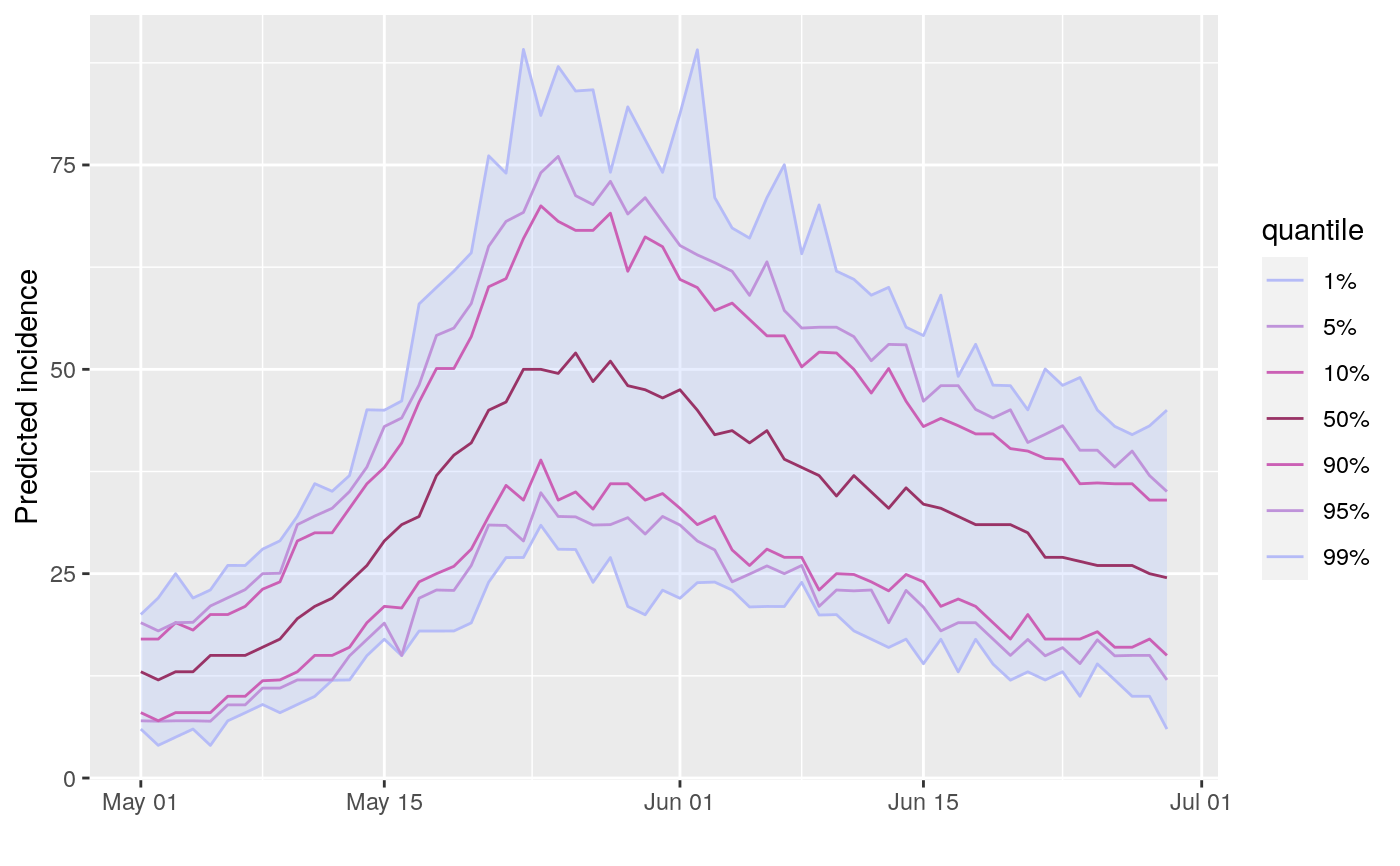This function simulates future incidence based on past incidence data, a selection of plausible reproduction numbers (R), and the distribution of the serial interval (time from primary onset to secondary onset).

project(
x,
R,
si,
n_sim = 100,
n_days = 7,
R_fix_within = FALSE,
model = c("poisson", "negbin"),
size = 0.03,
time_change = NULL
)

## Arguments

x An incidence object containing daily incidence; other time intervals will trigger an error. A vector of numbers representing plausible reproduction numbers; for instance, these can be samples from a posterior distribution using the earlyR or EpiEstim packages. If time_change is provided, then it must be a vector (for fixed values of R per time window) or a list of vectors (for separate distributions of R per time window), with one element more than the number of dates in time_change. A function computing the serial interval, or a numeric vector providing its mass function, starting a day 1, so that si[i] is the PMF for serial interval of i. The model implicitly assumes that si = 0. For functions, we strongly recommend using the RECON package distcrete to obtain such distribution (see example). The number of epicurves to simulate. Defaults to 100. The number of days to run simulations for. Defaults to 14. A logical indicating if R should be fixed within simulations (but still varying across simulations). If FALSE, R is drawn for every simulation and every time step. Fixing values within simulations favours more extreme predictions (see details) Distribution to be used for projections. Must be one of "poisson" or "negbin" (negative binomial process). Defaults to poisson size parameter of negative binomial distribition. Ignored if model is poisson an optional vector of times at which the simulations should use a different sample of reproduction numbers, provided in days into the simulation (so that day '1' is the first day after the input incidence object); if provided, n dates in time_change will produce n+1 time windows, in which case R should be a list of vectors of n+1 R values, one per each time window.

## Details

The decision to fix R values within simulations (R_fix_within) reflects two alternative views of the uncertainty associated with R. When drawing R values at random from the provided sample, (R_fix_within set to FALSE), it is assumed that R varies naturally, and can be treated as a random variable with a given distribution. When fixing values within simulations (R_fix_within set to TRUE), R is treated as a fixed parameter, and the uncertainty is merely a consequence of the estimation of R. In other words, the first view is rather Bayesian, while the second is more frequentist.

## Examples


## example using simulated Ebola outbreak
if (require(outbreaks) &&
require(distcrete) &&
require(incidence) &&
require(magrittr)) {

si <- distcrete("gamma", interval = 1L,
shape = 2.4,
scale = 4.7,
w = 0.5)

i <- incidence(ebola_sim$linelist$date_of_onset)
plot(i)

## projections after the first 100 days, over 60 days, fixed R to 2.1

set.seed(1)
proj_1 <- project(x = i[1:100], R = 2.1, si = si, n_days = 60)
plot(proj_1)

## add projections to incidence plot
plot(i[1:160]) %>% add_projections(proj_1)

## projections after the first 100 days, over 60 days,
## using a sample of R

set.seed(1)
R <- rnorm(100, 1.8, 0.2)
hist(R, col = "grey", border = "white", main = "Distribution of R")
proj_2 <- project(x = i[1:100], R = R, si = si, n_days = 60)

## add projections to incidence plot
plot(i[1:160]) %>% add_projections(proj_2)

## same with R constant per simulation (more variability)

set.seed(1)
proj_3 <- project(x = i[1:100], R = R, si = si, n_days = 60,
R_fix_within = TRUE)

## add projections to incidence plot
plot(i[1:160]) %>% add_projections(proj_3)

## time-varying R, 2 periods, R is 2.1 then 0.5
set.seed(1)
proj_4 <- project(i,
R = c(2.1, 0.5),
si = si,
n_days = 60,
time_change = 40,
n_sim = 100)
plot(proj_4)

## time-varying R, 2 periods, separate distributions of R for each period
set.seed(1)
R_period_1 <- runif(100, min = 1.1, max = 3)
R_period_2 <- runif(100, min = 0.6, max = .9)

proj_5 <- project(i,
R = list(R_period_1, R_period_2),
si = si,
n_days = 60,
time_change = 20,
n_sim = 100)
plot(proj_5)

}#> Scale for 'x' is already present. Adding another scale for 'x', which will
#> replace the existing scale.#> Scale for 'x' is already present. Adding another scale for 'x', which will
#> replace the existing scale.#> Scale for 'x' is already present. Adding another scale for 'x', which will
#> replace the existing scale.